# 數方

﻿﻿﻿﻿﻿﻿﻿﻿﻿﻿﻿﻿﻿﻿﻿﻿﻿﻿﻿﻿﻿﻿﻿﻿﻿﻿

Special thanks to aa124816 for the translation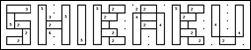Q. How to mark a rectangle?
A. It is enough to start marking the rectangle at the middle of the start square and release the mouse at the somewhere inside the opposite square. Something like this: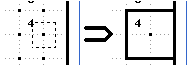Q. How to remove a line:
A. You should surround the lines you want removed with the red marking tool. That happens when you use the right mouse button:Q. How to solve a puzzle:
A. Here is an example of how a puzzle is solved: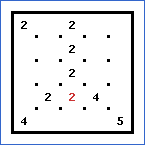First we notice that the only rectangle with 2 squares that can fit the red 2 is this one: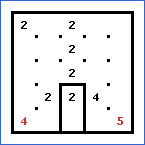Then notice the red 4 and 5 in the bottom corners. Their rectangles can be only the following: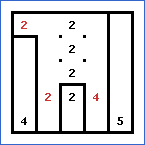The red 2 in the top-left corner is analogical to the first one.  The squares below the bottom red 2 and 4 should definitely be part of their rectangles since there cannot be a rectangle without a number. So this is the result: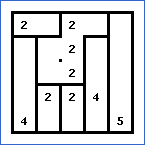It is quite easy to finish the puzzle from this point: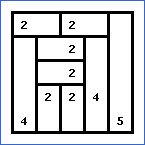zh.puzzle-shikaku.com